Download Presentation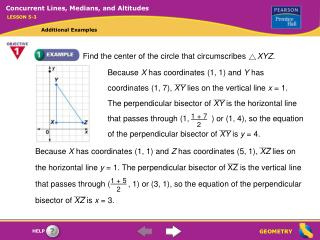Find the center of the circle that circumscribes XYZ .

# Find the center of the circle that circumscribes XYZ . - PowerPoint PPT Presentation

Find the center of the circle that circumscribes XYZ . Because X has coordinates (1, 1) and Y has coordinates (1, 7), XY lies on the vertical line x = 1. The perpendicular bisector of XY is the horizontal line that passes through (1, ) or (1, 4), so the equationI am the owner, or an agent authorized to act on behalf of the owner, of the copyrighted work described.
Download Presentation## Find the center of the circle that circumscribes XYZ .

An Image/Link below is provided (as is) to download presentation

Download Policy: Content on the Website is provided to you AS IS for your information and personal use and may not be sold / licensed / shared on other websites without getting consent from its author.While downloading, if for some reason you are not able to download a presentation, the publisher may have deleted the file from their server.

- - - - - - - - - - - - - - - - - - - - - - - - - - E N D - - - - - - - - - - - - - - - - - - - - - - - - - -
Presentation Transcript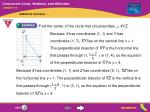Find the center of the circle that circumscribes XYZ.

Because X has coordinates (1, 1) and Y has

coordinates (1, 7), XY lies on the vertical line x = 1.

The perpendicular bisector of XY is the horizontal line

that passes through (1, ) or (1, 4), so the equation

of the perpendicular bisector of XY is y = 4.

1 + 7

2

Because X has coordinates (1, 1) and Z has coordinates (5, 1), XZ lies on

the horizontal line y = 1. The perpendicular bisector of XZ is the vertical line

that passes through ( , 1) or (3, 1), so the equation of the perpendicular

bisector of XZ is x = 3.

1 + 5

2

Concurrent Lines, Medians, and Altitudes

LESSON 5-3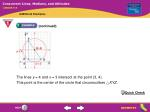The lines y = 4 and x = 3 intersect at the point (3, 4).

This point is the center of the circle that circumscribes XYZ.

Concurrent Lines, Medians, and Altitudes

LESSON 5-3

(continued)

Quick Check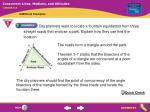The roads form a triangle around the park.

Concurrent Lines, Medians, and Altitudes

LESSON 5-3

City planners want to locate a fountain equidistant from three straight roads that enclose a park. Explain how they can find the location.

Theorem 5-7 states that the bisectors of the

angles of a triangle are concurrent at a point

equidistant from the sides.

The city planners should find the point of concurrency of the angle

bisectors of the triangle formed by the three roads and locate the

fountain there.

Quick Check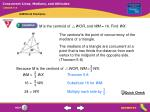M is the centroid of WOR, and WM = 16. Find WX.

2

3

Because M is the centroid of WOR, WM = WX.

2

3

WM = WXTheorem 5-8

2

3

16 = WXSubstitute 16 for WM.

3

2

24 = WXMultiply each side by .

Concurrent Lines, Medians, and Altitudes

LESSON 5-3

The centroid is the point of concurrency of the

medians of a triangle.

The medians of a triangle are concurrent at a

point that is two thirds the distance from each

vertex to the midpoint of the opposite side.

(Theorem 5-8)

Quick Check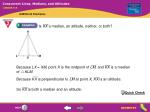Is KX a median, an altitude, neither, or both?

Because LX = XM, point X is the midpoint of LM, and KX is a median

of KLM.

Because KX is perpendicular to LM at point X, KX is an altitude.

So KX is both a median and an altitude.

Concurrent Lines, Medians, and Altitudes

LESSON 5-3